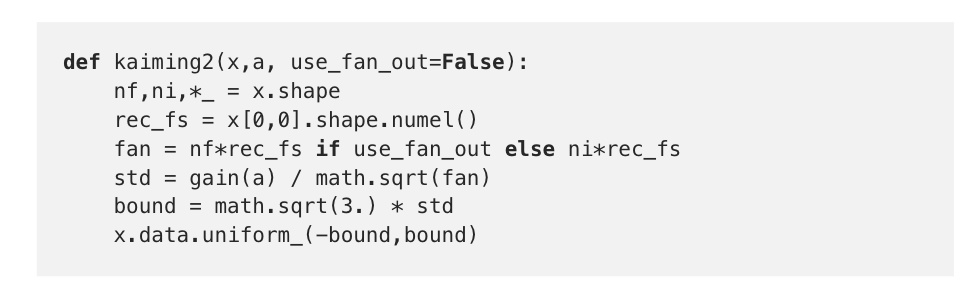# Kaiming Init — A Consolidation (fastai)

Original article can be found here (source): Deep Learning on Medium`# Kaiming Init for convolutional layerdef gain(a): return math.sqrt(2.0 / (1 + a**2))def kaiming2(x,a, use_fan_out=False): nf,ni,*_ = x.shape rec_fs = x[0,0].shape.numel() fan = nf*rec_fs if use_fan_out else ni*rec_fs std = gain(a) / math.sqrt(fan) bound = math.sqrt(3.) * std x.data.uniform_(-bound,bound)`

To relate code to paper, I first did a read-through of the relevant section in the paper (2.2. Initialization of Filter Weights for Rectifiers). I was quickly bogged down by the math and searched YouTube hoping for a video explanation. I came across Andrew Ng’s video called Weight Initialization in a Deep Network.